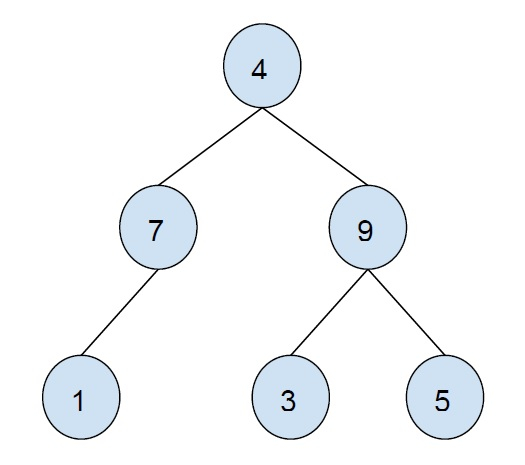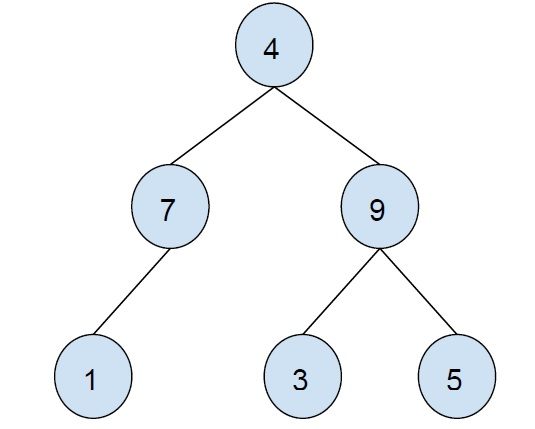# Print all internal nodes of a Binary tree in C++

In this problem, we are given a binary tree and we have to print all internal nodes of the binary tree.

The binary tree is a tree in which a node can have a maximum of 2 child nodes. Node or vertex can have no nodes, one child or two child nodes.

ExampleInternal Node is a node that can have at least one child i.e. non-leaf node is an internal node.

Let’s take an example to understand the problem −Output − 7 4 9

To solve this problem, we will traverse the binary tree using BFS(breadth-first search) traversal.

While traversal we will push nodes to a queue. When we pop elements from the queue, we will print all nodes of the tree that do not have any child nodes.

## Example

Our logic is implemented by the below code −

Live Demo

#include <bits/stdc++.h>
using namespace std;
struct Node {
int data;
Node *left, *right;
Node(int data){
left = right = NULL;
this->data = data;
}
};
void printNonLeafNodes(Node* root) {
queue<Node*> treeNodes;
treeNodes.push(root);
while (!treeNodes.empty()) {
Node* curr = treeNodes.front();
treeNodes.pop();
bool isInternal = 0;
if (curr->left) {
isInternal = 1;
treeNodes.push(curr->left);
}
if (curr->right) {
isInternal = 1;
treeNodes.push(curr->right);
}
if (isInternal)
cout<<curr->data<<"\t";
}
}
int main() {
Node* root = new Node(43);
root->left = new Node(12);
root->right = new Node(78);
root->left->left = new Node(4);
root->right->left = new Node(9);
root->right->right = new Node(1);
root->right->right->right = new Node(50);
root->right->right->left = new Node(25);
cout<<"All internal Nodes of the binary tree are :\n";
printNonLeafNodes(root);
return 0;
}

## Output

All internal Nodes of the binary tree are −
43 12 78 1